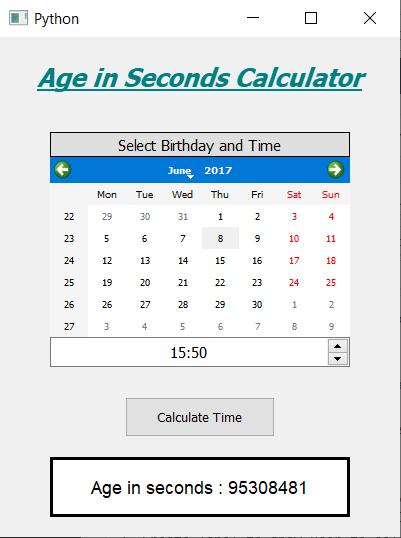# PyQt5 – Calculator for Number of seconds You have Lived

In this article we will see how we can create calculator which tells how many seconds a person has lived. Below si how the calculator will look likeConcept : We can get the age in seconds by getting the difference between the birth date and the birth time with the present date and time with the help of python datetime objects, which will keep count of leap years and we can get the number of days and seconds between the dates. Then these days can be converted into seconds to get the total seconds count.

GUI Implementation Steps :
1. Create a heading label that display the calculator name
2. Create label to show user to select the birth date and the birth time
3. Create a QCalendarWidget object for user to select the birth date
4. Create a QTimeEdit object to get the birth time
5. Create a push button to calculate the age in seconds
6. Create a label to show the calculated age in seconds

Back-End Implementation :
1. Make the calendar future date block i.e set current date as maximum date
2. Add action to the push button
3. Inside the push button action get the date from the calendar and time from the QTimeEdit
4. Get the day, month and year from the date and hour and minute from the time edit
5. Create a datetime object for current date and the birth date
6. Get the difference from both the dates and get the days and seconds
7. Convert days into seconds and add it to the original seconds
8. Show the calculated seconds value through label

Below is the implementation

 `# importing libraries ` `from` `PyQt5.QtWidgets ``import` `*`  `from` `PyQt5 ``import` `QtCore, QtGui ` `from` `PyQt5.QtGui ``import` `*`  `from` `PyQt5.QtCore ``import` `*`  `import` `datetime ` `import` `sys ` ` `  ` `  `class` `Window(QMainWindow): ` ` `  `    ``def` `__init__(``self``): ` `        ``super``().__init__() ` ` `  `        ``# setting title ` `        ``self``.setWindowTitle(``"Python "``) ` ` `  `        ``# width of window ` `        ``self``.w_width ``=` `400` ` `  `        ``# height of window ` `        ``self``.w_height ``=` `500` ` `  `        ``# setting geometry ` `        ``self``.setGeometry(``100``, ``100``, ``self``.w_width, ``self``.w_height) ` ` `  `        ``# calling method ` `        ``self``.UiComponents() ` ` `  `        ``# showing all the widgets ` `        ``self``.show() ` ` `  `    ``# method for components ` `    ``def` `UiComponents(``self``): ` ` `  `        ``# creating head label ` `        ``head ``=` `QLabel(``"Age in Seconds Calculator"``, ``self``) ` ` `  `        ``head.setWordWrap(``True``) ` ` `  `        ``# setting geometry to the head ` `        ``head.setGeometry(``0``, ``10``, ``400``, ``60``) ` ` `  `        ``# font ` `        ``font ``=` `QFont(``'Times'``, ``15``) ` `        ``font.setBold(``True``) ` `        ``font.setItalic(``True``) ` `        ``font.setUnderline(``True``) ` ` `  `        ``# setting font to the head ` `        ``head.setFont(font) ` ` `  `        ``# setting alignment of the head ` `        ``head.setAlignment(Qt.AlignCenter) ` ` `  `        ``# setting color effect to the head ` `        ``color ``=` `QGraphicsColorizeEffect(``self``) ` `        ``color.setColor(Qt.darkCyan) ` `        ``head.setGraphicsEffect(color) ` ` `  `        ``# creating a label ` `        ``b_label ``=` `QLabel(``"Select Birthday and Time"``, ``self``) ` ` `  `        ``# setting properties  label ` `        ``b_label.setAlignment(Qt.AlignCenter) ` `        ``b_label.setGeometry(``50``, ``95``, ``300``, ``25``) ` `        ``b_label.setStyleSheet(``"QLabel"` `                              ``"{"` `                              ``"border : 1px solid black;"` `                              ``"background : rgba(70, 70, 70, 25);"` `                              ``"}"``) ` `        ``b_label.setFont(QFont(``'Times'``, ``9``)) ` ` `  `        ``# creating a calendar widget to select the date ` `        ``self``.calendar ``=` `QCalendarWidget(``self``) ` ` `  `        ``# setting geometry of the calendar ` `        ``self``.calendar.setGeometry(``50``, ``120``, ``300``, ``180``) ` ` `  `        ``# setting font to the calendar ` `        ``self``.calendar.setFont(QFont(``'Times'``, ``6``)) ` ` `  `        ``# getting current date ` `        ``date ``=` `QDate.currentDate() ` ` `  `        ``# blocking future dates ` `        ``self``.calendar.setMaximumDate(date) ` ` `  `        ``# creating a time edit object to receive time ` `        ``self``.time ``=` `QTimeEdit(``self``) ` ` `  `        ``# setting properties to the time ` `        ``self``.time.setGeometry(``50``, ``300``, ``300``, ``30``) ` `        ``self``.time.setAlignment(Qt.AlignCenter) ` `        ``self``.time.setFont(QFont(``'Times'``, ``9``)) ` ` `  ` `  `        ``# creating a push button ` `        ``calculate ``=` `QPushButton(``"Calculate Time"``, ``self``) ` ` `  `        ``# setting geometry to the push button ` `        ``calculate.setGeometry(``125``, ``360``, ``150``, ``40``) ` ` `  `        ``# adding action to the calculate button ` `        ``calculate.clicked.connect(``self``.calculate_action) ` ` `  `        ``# creating a label to show percentile ` `        ``self``.result ``=` `QLabel(``self``) ` ` `  `        ``# setting properties to result label ` `        ``self``.result.setAlignment(Qt.AlignCenter) ` `        ``self``.result.setGeometry(``50``, ``420``, ``300``, ``60``) ` `        ``self``.result.setWordWrap(``True``) ` `        ``self``.result.setStyleSheet(``"QLabel"` `                                  ``"{"` `                                  ``"border : 3px solid black;"` `                                  ``"background : white;"` `                                  ``"}"``) ` `        ``self``.result.setFont(QFont(``'Arial'``, ``11``)) ` ` `  ` `  `    ``def` `calculate_action(``self``): ` ` `  `        ``# getting birth date day ` `        ``birth ``=` `self``.calendar.selectedDate() ` ` `  `        ``# getting year and month day of birth day ` `        ``birth_year ``=` `birth.year() ` `        ``birth_month ``=` `birth.month() ` `        ``birth_day ``=` `birth.day() ` ` `  `        ``# getting time of from the time edit ` `        ``time ``=` `self``.time.time() ` ` `  `        ``# getting hour and seconds ` `        ``hour ``=` `time.hour() ` `        ``minute ``=` `time.minute() ` ` `  `        ``# getting today date ` `        ``current ``=` `QDate.currentDate() ` ` `  `        ``# getting year and month day of current day ` `        ``current ``=` `datetime.datetime.now() ` ` `  ` `  `        ``# coverting  date into date object ` `        ``birth_date ``=` `datetime.datetime(birth_year, birth_month, birth_day, hour, minute, ``0``) ` ` `  `        ``# getting difference in both the dates ` `        ``difference ``=` `current ``-` `birth_date ` ` `  `        ``# getting difference in days ` `        ``days ``=` `difference.days ` ` `  `        ``# setting seconds ` `        ``seconds ``=` `difference.seconds ` ` `  `        ``# converting days into second and adding them ` `        ``seconds ``=` `seconds ``+` `days ``*` `24` `*` `60` `*` `60` ` `  ` `  ` `  `        ``# setting this value with the help of label ` `        ``self``.result.setText(``"Age in seconds : "` `+` `str``(seconds)) ` ` `  `  `  ` `  `# create pyqt5 app ` `App ``=` `QApplication(sys.argv) ` ` `  `# create the instance of our Window ` `window ``=` `Window() ` ` `  `# start the app ` `sys.exit(App.``exec``()) `

Output :

My Personal Notes arrow_drop_upCheck out this Author's contributed articles.

If you like GeeksforGeeks and would like to contribute, you can also write an article using contribute.geeksforgeeks.org or mail your article to contribute@geeksforgeeks.org. See your article appearing on the GeeksforGeeks main page and help other Geeks.

Please Improve this article if you find anything incorrect by clicking on the "Improve Article" button below.

Article Tags :

Be the First to upvote.

Please write to us at contribute@geeksforgeeks.org to report any issue with the above content.